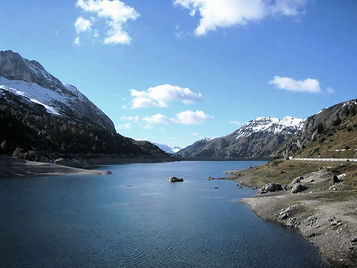## VAM: Effects of Gradient & Altitude

###### 19 Jun 2009

When evaluating the VAM of a certain climbing performance we need to consider wind, drafting, asphalt conditions but also average SLOPE GRADIENT of the climb and the ALTITUDES at the start and end of the ascent.

There is quite a difference between a VAM obtained on an average gradient of 10% than one with the same value but obtained over a climb at 7%.
Over the years I have been putting together and using a simple formula which is useful when comparing VAM's expressed over different gradients.
For example, a VAM=1800 m/h corresponds to a different value in watt/kg, depending on the average gradient of the climb:

- gradient 11% 1800/3.1 = 5.80 w/kg
- gradient 10% 1800/3.0 = 6.00 w/kg
- gradient 9% 1800/2.9 = 6.20 w/kg
- gradient 8% 1800/2.8 = 6.42 w/kg
- gradient 7% 1800/2.7 = 6.66 w/kg
- gradient 6% 1800/2.6 = 6.92 w/kg

It is therefore simply enough to subdivide the VAM value with a certain number, between 2.6 up to 3.1, in accordance with the steepness of the climb (from 6% to 11%).

At higher ALTITUDES, barometric pressure and the partial oxygen pressure (PpO2) reduce by about 6% every 500m of elevation.

A very interesting study (J.Appl.Physiol. 1996;80:2204-2210) verified in laboratory how 11 elite cyclists (VO2max = 77ml/kg/min) presented an average decrease in their VO2max by 6.8% at an altitude of 580 m, compared to sea level values.

It is therefore opportune to consider also the average altitude of the climb when comparing VAM's obtained at different elevations; the intrinsic values of a VAM done on a climb starting at 200m asl and ending at 1200m asl (average altitude of 700m) and one done on a climb starting at 1000m asl and ending at 1800m asl (average altitude of 1400m) will differ greatly.

A difference in average altitude of 700m means a reduction of PpO2 of 8%.
In reality the performance on the road will "only" decrease by about 5%, because of a more rarefied air that reduces air drag for the cyclist and friction in respiration (less dense air).

I think it is reasonable to evaluate an average reduction in VAM values by approximately 3% every 500m of elevation, while still considering individual variations due to efficiency in pulmonary gaseous exchanges.